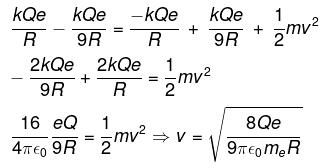Courses

# Test Level 3: Capacitance

## 18 Questions MCQ Test Physics Class 12 | Test Level 3: Capacitance

Description
This mock test of Test Level 3: Capacitance for Class 12 helps you for every Class 12 entrance exam. This contains 18 Multiple Choice Questions for Class 12 Test Level 3: Capacitance (mcq) to study with solutions a complete question bank. The solved questions answers in this Test Level 3: Capacitance quiz give you a good mix of easy questions and tough questions. Class 12 students definitely take this Test Level 3: Capacitance exercise for a better result in the exam. You can find other Test Level 3: Capacitance extra questions, long questions & short questions for Class 12 on EduRev as well by searching above.
QUESTION: 1

### A wheel having mass m has charges +q and -q on diametrically opposite points. It remains in equilibrium on a rough inclined plane in the presence of a uniform vertical electric field E. The value of E is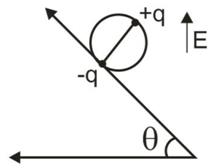Solution: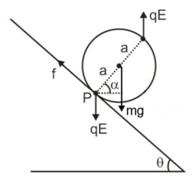For rotational equilibrium , ∑τp = 0
⇒ mga cos α  - qE (2a cos) = 0
⇒ E = mg/2q

*Multiple options can be correct
QUESTION: 2

### The two plates X and Y of a parallel plate capacitor of capacitance C are given a charge of amount Q each. X is now joined to the positive terminal and Y to the negative terminal of a cell of emf E = Q/C.

Solution:

As the emf of cell is ϵ=Q/C, charge of amount Q will flow from the positive terminal to the negative terminal of the cell through the capacitor.
As the Y is connected to the negative terminal of the cell so it is ground and the total charge on plate Y will be zero.
Here cell charge the capacitor plate X and its total charge =(Q+Q)=2Q
The energy will supply by cell is U=​Cϵ2

*Multiple options can be correct
QUESTION: 3

### Two capacitors of capacitances 1mF and 3mF are charged to the same voltages 5V. They are connected in parallel with oppositely charged plates connected together. Then

Solution:
*Multiple options can be correct
QUESTION: 4

Each plate of a parallel plate capacitor has a charge q on it. The capacitor is now connected to a battery. Now,

Solution:

(a) The facing surfaces of the capacitor have equal and opposite charges

(b) The two plates of the capacitor have equal and opposite charges

(d) The outer surfaces of the plates have equal charges

Explanation:

q-Q=q+QàQ=0

• Please note, option (c) is incorrect the reason being why is that the battery is a source of energy not charge.

• If a capacitor plates have equal charge on outside and equal charge on inside, then one can think that the charge on the plates must be equal so option (b) cannot be incorrect.

*Multiple options can be correct
QUESTION: 5

When a parallel plates capacitor is connected to a source of constant potential difference,

Solution:
*Multiple options can be correct
QUESTION: 6

When two identical capacitors are charged individually to different potentials and connected parallel to each other, after disconnecting them from the source :

Solution:
*Multiple options can be correct
QUESTION: 7

Two thin conducting shells of radii R and 3R are shown in the figure. The outer shell carries a charge +Q and the inner shell is neutral. The inner shell is earthed with the help of a switch S.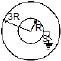Solution:

(A)  Potential of inner shell is due to potential developed by outer shell.
(B)   Since the inner shell is Earthed so its potential becomes zero.
(C)  Vinner=(KQ/3R)+(KQI/R)=0⇒rQ'=−Q/3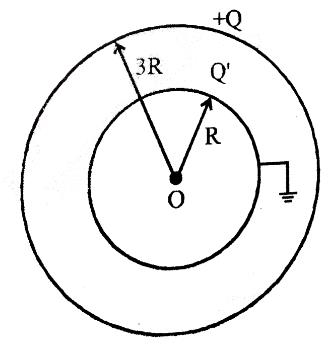(D) It capacitance becomes =(4π∈0R1R2/R2−R1)+4π∈R2

*Multiple options can be correct
QUESTION: 8

Two capacitors of 2mF and 3mF are charged to 150 volt and 120 volt respectively. The plates of capacitor are connected as shown in the figure. A discharged capacitor of capacity 1.5 mF falls to the free ends of the wire. Then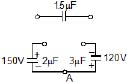Solution:

Charge in 2μF Capacitor =300μC
Charge in 3μF Capacitor =360μC
when 1.5μF falls on the circuit ends, Redistribution of charge now will be:
Charge in 2μF Capacitor =300−xμC
Charge in 3μF Capacitor =360−xμC
Charge in 1.5μF Capacitor =xμC
By Kirchhoff Voltage Law:
x/1.5=[(300−x​)/2]+[(360−x)/3]​
which gives x=180μC
Therefore, Answers are A, B & C

*Multiple options can be correct
QUESTION: 9

In the circuit shown initially C1, C2 are uncharged. After closing the switch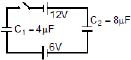Solution:

Here C1​ and C2​ are in series and the total potential between the capacitors is VT​=12+6=18V
Ceq​=4×8/4+8 ​=(8/3)​μF and
Qeq​=Ceq​VT​=(8/3)×18=48μC
As they are in series so the charge on each capacitor is equal to Qeq​=48μC.
∴Q1​=Q2​=48μC
Potential across C1​ is V1​= Q1/C1​ ​​=48/4​=12V
Potential across C2​ is V2​= Q2/C2​ ​​=48/8​=6V

*Multiple options can be correct
QUESTION: 10

A circuit shown in the figure consists of a battery of emf 10V and two capacitance Cand C2 of capacitances 1.0mF and 2.0mF respectively. The potential difference VA – VB is 5V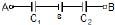Solution:

VA−q1+10−(q/2)=VBVA-q1+10-q2=VB
VA−VB+10=(q/1)+q2VA-VB+10=q1+q2
5+10=3q/2⇒q=10μC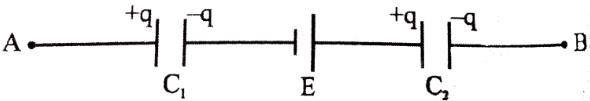V1=10μC/1μF=10,& V2=10μC/2μF=5V
U1=q2/(2×C1),&U2=q2/2C2
Since C2=2C1 so U1=2U2

*Multiple options can be correct
QUESTION: 11

Two capacitors of equal capacitance (C1 = C2) are shown in the figure. Initially, while the switch S is open, one of the capacitors is uncharged and the other carries charge Q0. The energy stored in the charged capacitor is U0. Sometimes after the switch is colsed, the capacitors C1 and C2 carry charges Q1 and Q2, respectively, the voltages across the capacitors are V1 and V2, and the energies stored in the capacitors are U1 and U2. Which of the following statements is INCORRECT ?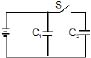Solution:
*Multiple options can be correct
QUESTION: 12

Identify the correct statements.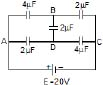Solution:
QUESTION: 13

A solid conducting sphere of radius 10 cm is enclosed by a thin metallic shell of radius 20 cm. A charge q = 20 mC is given to the inner sphere. Find the heat generated in the process, the inner sphere is connected to the shell by a conducting wire -

Solution:

On connecting, the entire amount of charge will shift to the outer sphere. Heat generated is
Ui​−Uf​= (q2/8πε0​R1​) – (q2​/8πε0​R2​)
=[(20×10−6)×9×109/2] ​[(1/0.10)​−10.20]=9J

QUESTION: 14

The plates of a parallel plate capacitor are given charges +4Q and _2Q. The capacitor is then connected across an uncharged capacitor of same capacitance as first one (= C). Find the final potential difference between the plates of the first capacitor.

Solution:

Based on the symmetry of charges at equilibrium condition, +4Q will divide into −2Q each. Similarly−2Q will divide into −Q each.
Effectively capacitance=C+C=2C
So, the final potential difference = 2Q−(Q)​/ 2C =3Q​/2C

QUESTION: 15

Three capacitors of 2 µF, 3 µF and 5 µF are independently charged with batteries of emf’s 5V, 20V and 10V respectively. After disconnecting from the voltage source. These capacitors are connected as shown in figure with their positive polarity plates connected to A and negative polarity is earthed. Now a battery of 20V and an uncharged capacitor of 4 µF capacitance are connected to the junction A as shown with a switch S. when switch is closed, find: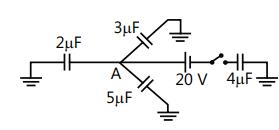(a) The potential of the junction A.

(b) Final charge on all four capacitros.

Solution: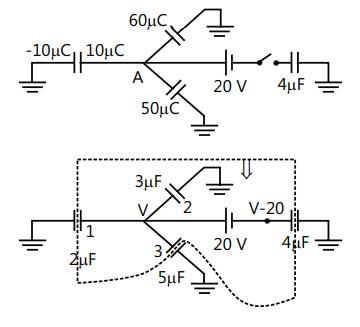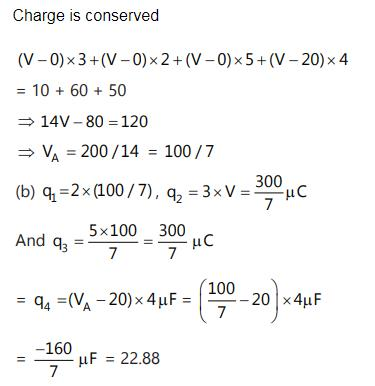QUESTION: 16

Positive mutual potential energy of a system containing two charges corresponds to

Solution:
QUESTION: 17

A charge q = 1.0 C moves distance of 1.5 m in the direction of a uniform electric field E of magnitude 2.0 N/C. Find its change in electrostatic potential energy.

Solution:

△U=?
△U=q△v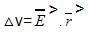=Ercosθ
=2x1.5
=3v
Therefore, △U=1x3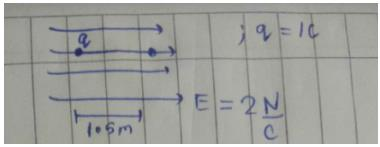QUESTION: 18

Two uniformly charged nonconducting spheres, each of radius R, are fixed in a gravity free space as shown in the figure. If an electron is released at rest from the point A, then its speed just before striking the other sphere is [mass of electron - me]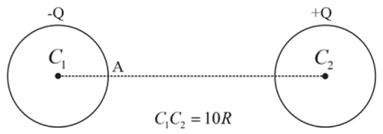Solution:

using the conservation of mechanical energy, we get